# acceleration

(redirected from Accelerated motion)
Also found in: Dictionary, Thesaurus, Medical, Legal, Wikipedia.
Related to Accelerated motion: Uniform motion, Uniformly accelerated motion

## acceleration,

change in the velocityvelocity,
change in displacement with respect to time. Displacement is the vector counterpart of distance, having both magnitude and direction. Velocity is therefore also a vector quantity. The magnitude of velocity is known as the speed of a body.
of a body with respect to time. Since velocity is a vectorvector,
quantity having both magnitude and direction; it may be represented by a directed line segment. Many physical quantities are vectors, e.g., force, velocity, and momentum.
quantity, involving both magnitude and direction, acceleration is also a vector. In order to produce an acceleration, a forceforce,
commonly, a "push" or "pull," more properly defined in physics as a quantity that changes the motion, size, or shape of a body. Force is a vector quantity, having both magnitude and direction.
must be applied to the body. The magnitude of the force F must be directly proportional to both the mass of the body m and the desired acceleration a, according to Newton's second law of motion, F=ma. The exact nature of the acceleration produced depends on the relative directions of the original velocity and the force. A force acting in the same direction as the velocity changes only the speedspeed,
change in distance with respect to time. Speed is a scalar rather than a vector quantity; i.e., the speed of a body tells one how fast the body is moving but not the direction of the motion.
of the body. An appropriate force acting always at right angles to the velocity changes the direction of the velocity but not the speed. An example of such an accelerating force is the gravitational force exerted by a planet on a satellite moving in a circular orbit. A force may also act in the opposite direction from the original velocity. In this case the speed of the body is decreased. Such an acceleration is often referred to as a deceleration. If the acceleration is constant, as for a body falling near the earth, the following formulas may be used to compute the acceleration a of a body from knowledge of the elapsed time t, the distance s through which the body moves in that time, the initial velocity vi, and the final velocity vf:
a=(vf2vi2)/2s
a=2(svit)/t2
a=(vfvi)/t

## Acceleration

The time rate of change of velocity. Since velocity is a directed or vector quantity involving both magnitude and direction, a velocity may change by a change of magnitude (speed) or by a change of direction or both. It follows that acceleration is also a directed, or vector, quantity. If the magnitude of the velocity of a body changes from v1 ft/s to v2 ft/s in t seconds, then the average acceleration a has a magnitude given by Eq. (1):

(1)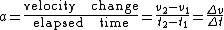To designate it fully the direction should be given, as well as the magnitude. See Velocity

Instantaneous acceleration is defined as the limit of the ratio of the velocity change to the elapsed time as the time interval approaches zero. When the acceleration is constant, the average acceleration and the instantaneous acceleration are equal.

Whenever a body is acted upon by an unbalanced force, it will undergo acceleration. If it is moving in a constant direction, the acting force will produce a continuous change in speed. If it is moving with a constant speed, the acting force will produce an acceleration consisting of a continuous change of direction. In the general case, the acting force may produce both a change of speed and a change of direction.

Angular acceleration is a vector quantity representing the rate of change of angular velocity of a body experiencing rotational motion. If, for example, at an instant t1, a rigid body is rotating about an axis with an angular velocity ω1, and at a later time t2, it has an angular velocity ω2, the average angular acceleration α is given by Eq. (2),

(2)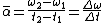in radians per second per second. The instantaneous angular acceleration is given by α = dω/dt.

When a body moves in a circular path with constant linear speed at each point in its path, it is also being constantly accelerated toward the center of the circle under the action of the force required to constrain it to move in its circular path. This acceleration toward the center of path is called radial acceleration. The component of linear acceleration tangent to the path of a particle subject to an angular acceleration about the axis of rotation is called tangential acceleration. See Rotational motion

McGraw-Hill Concise Encyclopedia of Physics. © 2002 by The McGraw-Hill Companies, Inc.

## acceleration

1. (linear acceleration) Symbol: a . The rate of increase of velocity with time, measured in meters per second per second (m s–2), etc.
2. (angular acceleration) Symbol: α. The rate of increase of angular velocity with time, usually measured in radians per second per second.
Collins Dictionary of Astronomy © Market House Books Ltd, 2006
The following article is from The Great Soviet Encyclopedia (1979). It might be outdated or ideologically biased.

## Acceleration

(1) In biological evolution, an acceleration of the development of some parts of the embryo at a definite stage of development. The Russian scientist A. N. Severtsov used the term acceleration to describe a situation in which a particular organ appears earlier in the ontogenesis and develops faster than other organs; as a result this organ begins functioning when the conditions of life make it necessary. Examples of acceleration include the earlier evolution of the mouth apparatus of fish and all ecaudate amphibians, which makes possible their feeding after their reserves of yolk have been exhausted; the evolution of milk teeth among mammals; and changes in the nature of fins and the development of vertebral arches and ribs among some fish when these bone elements are formed, bypassing the stage of cartilaginous skeleton formations.

L. IA. BLIAKHER

(2) In anthropology the more rapid somatic development and physiological maturation of children in adolescence that has been observed in the past 100–150 years; in a wider sense it refers to changes in bodily measurements and rates of somatic development in the course of a man’s whole life cycle. Acceleration occurs among all social groups of the population and refers to a variety of anatomical and physiological indications. Sometimes the term psychological acceleration is used in the literature to refer to accelerated mental development, although there is as yet no reliable statistical material on the subject. Changes in bodily measurement have been better studied than other areas. The size of children at birth is on the average 0.5–1 cm greater and the weight 100–300 grams greater than 100 years ago. Between 1880 and 1950 the height of children ages 5–7 increased by 1.5 cm every 10 years and the weight by 0.5 kg. The height of school-age children rose 10–15 cm in the same period. The age of sexual maturation has dropped an average of from one to two years in the past 75–100 years. Thus, the average of the first menstruation in Europe dropped from 16.5 years in the early 19th century to 13–13.5 years. The climacteric sets on not at 43–45 years, as was the case in the early 20th century, but at 48–50 years.

There are many hypotheses on the causes of acceleration: better food, development of hygiene, the neurogenic hypothesis (which explains acceleration in big cities as the action of urban stimuli on the nervous system); and several endogenous hypotheses (for instance, one that explains acceleration through the greater number of marriages between people from distant places). But all these hypotheses are one-sided and open to criticism. Most likely, acceleration is caused by many biological and social factors. The significance of acceleration for mankind still has not been studied.

### REFERENCES

Bunak, V. V. “Ob uvelichenii rosta i uskorenii polovogo sozrevaniia sovremennoi molodezhi v svete sovetskikh somatologicheskikh issledovanii.” Voprosy antropologii, 1968, issue 28.
Solovieva, V. S. “Obzor nekotorykh gipotez o prichinakh aktseleratsii.” Voprosy antropologii, 1967, issue 26.
Tanner, J. M. Growth at Adolescence. Oxford, 1962.

V. S. SOLOV’EVA and N. N. MIKLASHEVSKAIA

## Acceleration

a vector quantity characterizing the rate at which the velocity of a point changes in magnitude and direction with respect to time. If the point is in rectilinear motion and its velocity v increases or decreases uniformly, the magnitude of the acceleration is w = Δvt, where Δv is the change in velocity in the time interval Δt. In the general case the acceleration vector w is the first derivative of the velocity vector v with respect to time: w = dv/dt = . The acceleration vector is directed inward on the concave side of the point’s path and lies in the osculating plane.

In a rectangular Cartesian coordinate system the projections of acceleration on the x-axis, y-axis, and z-axis are equal to the first derivatives of the projections of velocity, or to the second derivatives of the coordinates of the point, with respect to time: wx = x, wy = y = ÿ, and wz = z = . Moreover, the absolute value of acceleration is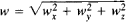At each point on the path of motion the acceleration has a tangential component wT and a normal, or centripetal, component wn; the former is the projection of the acceleration on the tangent to the path, and the latter is the projection of the acceleration on the principal normal to the path. The two components are defined by the equations wτ = dv/dt = and wn = v2, where v is the magnitude of the velocity and ρ is the radius of curvature of the path at the point in question. It can be shown that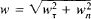The tangential component characterizes the change in the magnitude of the velocity, and the normal component characterizes the change in the direction of the velocity.

The relation among the acceleration of a free material particle, the particle’s mass m, and the applied force F is given by Newton’s second law: mw = F. The dimensions of acceleration are LT–2.

The acceleration of points of a rotating body is discussed in ROTATIONAL MOTION OF RIGID BODIES and ANGULAR ACCELERATION.

S. M. TARG

Physiological effects. According to the character of the effects on an organism, three types of acceleration are distinguished: impact linear acceleration, here defined as acceleration having a duration of not more than 1 sec and a rate of onset of not less than 10 g/sec (1 g is the acceleration of gravity at sea level); sustained linear acceleration, here defined as acceleration lasting not less than 1 sec and having a rate of onset of not more than 10 g/sec; and angular acceleration. In aviation and space medicine the “increased weight” of the body owing to acceleration is characterized by what is called the g factor.

A person is subjected to the greatest impact linear accelerations in falls, transportation accidents, the emergency landing of an airplane or spacecraft, ejection, and so on. The principal adverse pathophysiological effect of impact acceleration is damage to organs and tissues (spine, skull, and internal organs). The tolerance to impact accelerations directed perpendicularly to the body’s long axis is approximately twice as great as the tolerance to accelerations directed along the long axis; the respective tolerances are 30–40 g and 15–20 g. In the course of evolution man developed specific mechanisms of protection against impact acceleration, such as the shock-absorbing properties of the bone-support apparatus and the suspension system of the internal organs.

The extent of the adverse effect of sustained linear acceleration depends on the magnitude of the acceleration and its direction relative to the person’s body. The more closely the acceleration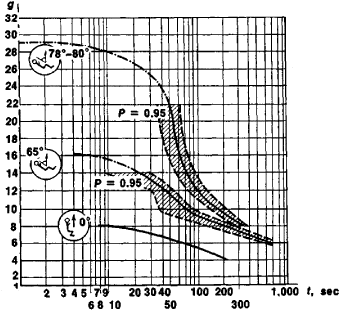Figure 1. Man’s tolerance time to sustained accelerations as a function of their magnitude and direction: (P) confidence interval for a probability of 0.95

vector approaches the body’s long axis and the direction of the main blood vessels, the more pronounced are the disruptions of circulation associated with the redistribution of blood due to increased hydrostatic pressure. Accelerations that lead to an increase in the amount of blood in vessels in the head are tolerated most poorly. A person withstands sustained acceleration best when the acceleration vector forms an angle of 75°–80° with the long axis of the body (see Figure 1). This condition is met on spacecraft of the Soyuz and Apollo types. The greatest sustained linear accelerations that a person may be subjected to at the present time occur during the maneuvering of a high-speed airplane or during the flight of a spacecraft along a ballistic trajectory. In the course of evolution man had practically no encounters with sustained linear acceleration. The tolerance to such acceleration is determined by general, nonspecific mechanisms of adaptation to adverse factors in the environment.

Rotary motions involve angular accelerations, which exert a specific influence on the vestibular apparatus and at certain values may bring about effects characteristic of impact and sustained linear acceleration.

Tolerance to acceleration is increased through the use of various types of equipment to maintain the person’s optimal posture and position with respect to the acceleration vector, to lower the magnitude and rate of increase of the acceleration, and to reduce the effects of blood redistribution in the organism. Such equipment includes shock-absorbing, form-fitting seats, safety belts, crash helmets, and G suits.

### REFERENCES

Barer, A. S. “Problemy uskorenii v kosmicheskoi fiziologii.” Kosmicheskaia biologiia i medilsina, 1967, issue 1.
Sergeev, A. A. Fiziologicheskie mekhanizmy deistviia uskorenii. Leningrad, 1967.
Kralkii spravochnik po kosmicheskoi biologii i meditsine, 2nd ed. Moscow, 1972.
Osnovy kosmicheskoi biologii i medilsiny: Sovmesmoe sovetskoamerikanskoe izdanie, vol. 2, book 1. Moscow, 1975.

A. S. BARER

## acceleration

[ak‚sel·ə′rā·shən]
(mechanics)
The rate of change of velocity with respect to time.
McGraw-Hill Dictionary of Scientific & Technical Terms, 6E, Copyright © 2003 by The McGraw-Hill Companies, Inc.

## acceleration

1. The rate of change of the velocity of a moving body.
2. The rate of change, esp. the quickening of the natural progress of a process, such as hardening, setting, or strength development of concrete.
McGraw-Hill Dictionary of Architecture and Construction. Copyright © 2003 by McGraw-Hill Companies, Inc.

## acceleration

the rate of increase of speed or the rate of change of velocity.
Collins Discovery Encyclopedia, 1st edition © HarperCollins Publishers 2005

## accelerator

(1) See particle accelerator.

(2) Speeding up the retrieval of Web pages. See Web page acceleration and CDN.

(4) Speeding up hardware. See graphics accelerator, video accelerator and accelerator card.

(5) A quick way to gain knowledge when reading text on a Web page. For example, after highlighting a word and clicking a button, an accelerator may provide a dictionary definition, a foreign language translation or the map of a street address.

(6) A key combination such as Alt-Shift-G that is used to activate a task. It provides a faster activation method than selecting from a menu. See also special function key.

(7) An incubator that expects to develop the company considerably faster than normal. See incubator.
Copyright © 1981-2019 by The Computer Language Company Inc. All Rights reserved. THIS DEFINITION IS FOR PERSONAL USE ONLY. All other reproduction is strictly prohibited without permission from the publisher.
References in periodicals archive ?
Explain accelerated motion as usual (without the aid of the corresponding Concept Map).
Show them the map about accelerated motion (Figure 15) as a synthesis of the information given.
He was trying to extend them to accelerated motion in order to take gravitational interactions into account.
Einstein's general theory of relativity makes an essential connection among the force of gravity, the curvature of space and accelerated motions. One of the consequences of this connection is that frames of reference anchored on an object in accereated motion will appear to "drag," to lag or precess with respect to a frame fixed on bodies that are not accelerated.

Site: Follow: Share:
Open / Close